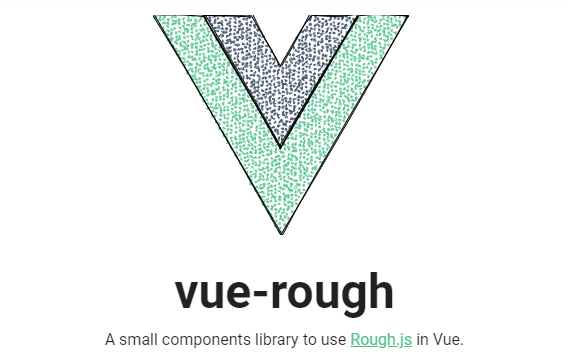/ Drawing

# A small components library to use Rough.js in Vue## vue-rough

A small components library to use Rough.js in Vue.

## Installation

``````\$ npm install vue-rough
``````
``````import VueRough from 'vue-rough';

Vue.use(VueRough);
``````

## Container Components

These wrap around visual components. You can choose between canvas or SVG as the container format.

``````<rough-canvas
width="500px"
height="500px"
:options="options"
></rough-canvas>
``````
``````<rough-svg
width="500px"
height="500px"
:options="options"
></rough-svg>
``````

`options` takes an Object with properties to be applied in general to all included visual components.
See the Rough.js wiki for a list of available
options.

## Visual Components

Shown below is a quick overview of the available components with their required properties.
All components may use additional properties. See the Rough.js wiki
for a full list of options. Components must be placed inside `rough-canvas` or `rough-svg`

### rough-line

``````<rough-line
:x1="1"
:y1="1"
:x2="20"
:y2="20"
/>
``````

### rough-rectangle

``````<rough-rectangle
:x1="20"
:y1="20"
:x2="40"
:y2="40"
/>
``````

### rough-ellipse

``````<rough-ellipse
:x="20"
:y="20"
:width="40"
:height="40"
/>
``````

### rough-circle

``````<rough-circle
:x="80"
:y="80"
:diameter="40"
/>
``````

### rough-linear-path

``````<rough-linear-path
:points="[[80, 10], [120, 20], [80, 120], [80, 100]]"
/>
``````

### rough-polygon

``````<rough-polygon
:vertices="[[390, 130], [490, 140], [350, 240], [490, 220]]"
/>
``````

### rough-arc

``````<rough-arc
:x="350"
:y="300"
:width="200"
:height="180"
:start="Math.PI / 2"
:stop="Math.PI"
:closed="true"
/>
``````

### rough-curve

``````<rough-curve
:points="[[10,328],[30,373],[50,390],[70,373],[90,328],[110,272],[130,227],[150,210],[170,227],[190,272],[210,328],[230,373],[250,390],[270,373],[290,328],[310,272],[330,227],[350,210],[370,227],[390,272]]"
/>
``````

### rough-path

``````<rough-path
d="M0 0l128 220.8L256 0h-51.2L128 132.48 50.56 0H0z"
/>
``````